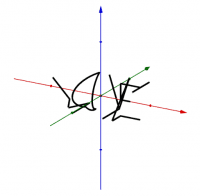# Customized Parametric Curves

Examples of parametric curves, created by single pairs of non-piecewise parametric equations. I developed this process for creating such curves (or at least if someone else beat me to it, I'm unaware). My process utilizes and reinforces high school level Math skills (domain/range, function transformations, function addition, function composition, writing sinusoid equations, parametric equations). Inspired by the "Batman Equation" of 2011 and Wolfram Alpha's "person curves." How-to article in NCTM's Mathematics Teacher: Learning and Teaching magazine, June 2020. https://www.nctm.org/Publications/Mathematics-Teacher_-Learning-and-Teaching-PK-12/ Instructional how-to video:
•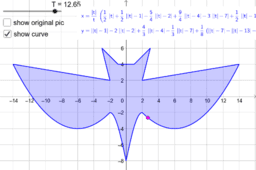### Parametric curve - Batbaby

•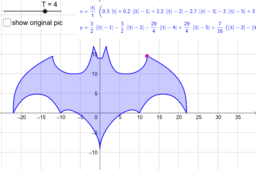### Parametric curve - Batman Begins

•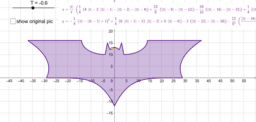### Parametric curve - Batman Dark Knight

•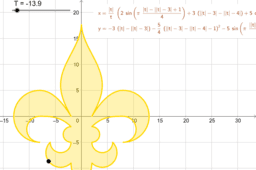### Parametric curve - Fleur de Lis

•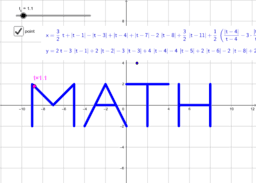### Parametric curve - MATH

•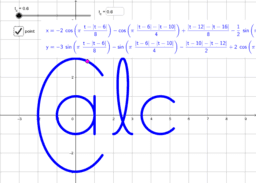### Parametric curve - CALC

•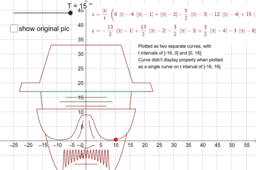### Parametric curve - Heisenberg

•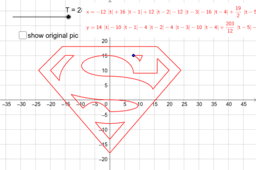### Parametric curve - Superman Logo

•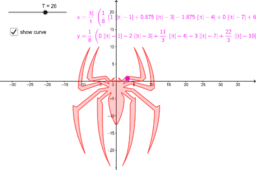### Parametric curve - Spiderman logo

•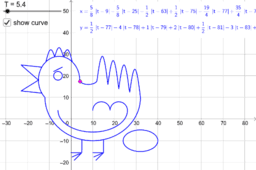### Parametric curve - Annoyed Chicken

•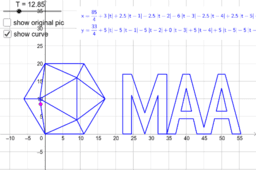### Parametric curve - MAA logo

•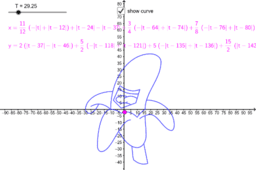### Parametric curve - Singing Banana logo

•### Parametric curve - Puzzle Ninja logo

•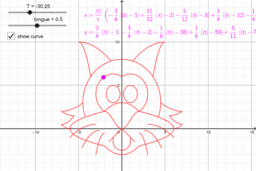### Parametric curve - Ambivalent Cat

•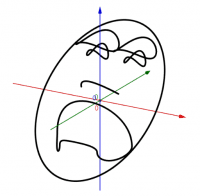### Parametric curve - 3D Comedy/Tragedy Face

•